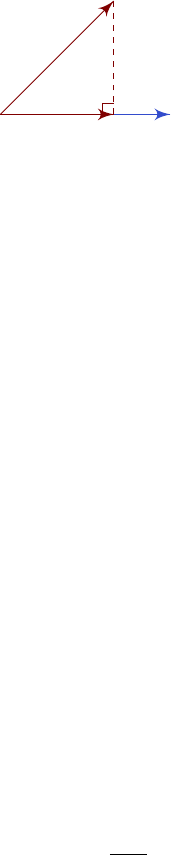2Vectors

IA Vectors and Matrices2.2 Scalar product
In a vector space, we can define the scalar product of two vectors, which returns
a scalar (i.e. a real or complex number). We will first look at the usual scalar
product defined for R
n
, and then define the scalar product axiomatically.
2.2.1 Geometric picture (R
2
and R
3
only)
Definition
(Scalar/dot product)
. a · b
=
|a||b|cos θ
, where
θ
is the angle
between a and b. It satisfies the following properties:
(i) a · b = b · a
(ii) a · a = |a|
2
0
(iii) a · a = 0 iff a = 0
(iv) If a · b = 0 and a, b 6= 0, then a and b are perpendicular.
Intuitively, this is the product of the parts of a and b that are parallel.
b
a
|a|
|a|cos θ
Using the dot product, we can write the projection of
b
onto
a
as (
|b|cos θ
)
ˆ
a
=
(ˆa · b)ˆa.
The cosine rule can be derived as follows:
|
BC|
2
= |
AC
AB|
2
= (
AC
AB) · (
AC
AB)
= |
AB|
2
+ |
AC|
2
2|
AB||
AC|cos θ
We will later come up with a convenient algebraic way to evaluate this scalar
product.
2.2.2 General algebraic definition
Definition
(Inner/scalar product)
.
In a real vector space
V
, an inner product
or scalar product is a map
V × V R
that satisfies the following axioms. It is
written as x ·y or hx | yi.
(i) x · y = y ·x (symmetry)
(ii) x · (λy + µz) = λx · y + µx ·z (linearity in 2nd argument)
(iii) x · x 0 with equality iff x = 0 (positive definite)
Note that this is a definition only for real vector spaces, where the scalars
are real. We will have a different set of definitions for complex vector spaces.
In particular, here we can use (i) and (ii) together to show linearity in 1st
argument. However, this is generally not true for complex vector spaces.
Definition. The norm of a vector, written as |a| or kak, is defined as
|a| =
a · a.
Example.